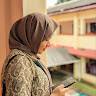The Joy of Programming
Positioning Shapes

We've seen how to draw circles of different sizes. However, the circles were always centered at the origin.

In this lesson we are going to focus on how to specify the center of the circle to position it at different places on the canvas.

## The Canvas

When we write a program to show some shapes, those shapes are displayed in a small box and that is called the canvas. It is of size 300x300 pixels and the point (0, 0) is at the center of the canvas.

You may be wondering what is pixels. Just like we measure length of physical objects in centimeters and inches, we measure things shown on the screen in pixels. Pixels are the dots that make up the screen. Our canvas is a square with 300 pixels length.

Here is an interactive demonstration to see how changing `x` and `y` changes the position of a circle. Change the sliders to change the position of the circle or click on any position to move the circle there.

## Positioning the Circle

We can specify the center of the circle using the `x` and `y` parameters.

``````c = circle(x=50, y=0, r=50)
show(c)
``````

Let us try to draw two circles next to each other.

``````c1 = circle(x=-50, y=0, r=50)
c2 = circle(x=50, y=0, r=50)
show(c1, c2)
``````

Wasn't that simple? Let's try some exercises now.

### Exercise 2.2: Three Circles in a Row

Draw three circles in a row as shown in the figure.

### Exercise 2.3: Three Circles in a Column

Draw three circles in a column as shown in the figure.

### Exercise 2.4: Grid of Circles

Draw grid of circles as shown in the figure.

### Exercise 2.5: Four Circles

Draw four circles as shows in the figure below.

### Exercise 2.6: Five Circles

Draw five circles as shows in the figure below.

### Exercise 2.7: Three Circles touching at the Bottom

Draw three circles with bottom point of each circle touching at the origin. The three circles will have radius of 25, 50 and 75 respectively.

### Exercise 2.8: Three Large Circles touching at the Bottom

Draw three circles with bottom point of each circle touching at the bottom most point of the canvas. The three circles will have radius of 50, 100 and 150 respectively.

Questions
AK
2 years ago
Post
Dismiss
Can you please explain how to do excercise 2.8 ie;three large circles touching at the bottom
Can you please explain how to do excercise 2.8 ie;three large circles touching at the bottom
DP
2 years ago
Post
Dismiss
c1= circle( x=0,y=50,r=50) c2= circle( x=0,y=100,r=100) c3= circle( x=0,y=150,r=150) show(c1,c2,c3) its the code but do it yourself after understanding the logic in it
c1= circle( x=0,y=50,r=50) c2= circle( x=0,y=100,r=100) c3= circle( x=0,y=150,r=150) show(c1,c2,c3) its the code but do it yourself after understanding the logic in it
VK
2 years ago
Post
Dismiss
`c1 = circle(x=0, y=-100, r=50) + circle(x=0,y=-50,r=100) + circle(x=0,y=0,r=150) show(c1)`
`c1 = circle(x=0, y=-100, r=50) + circle(x=0,y=-50,r=100) + circle(x=0,y=0,r=150) show(c1)`2 years ago
Post
Dismiss
c1 = circle(x=0, y=50, r=50) c2 = circle(x=0, y=100, r=100) c3 = circle(x=0, y=150, r=150) show(c1,c2,c3)
c1 = circle(x=0, y=50, r=50) c2 = circle(x=0, y=100, r=100) c3 = circle(x=0, y=150, r=150) show(c1,c2,c3)
JG
2 years ago
Post
Dismiss
c1=circle(x=0,y=50,r=50) show(c1) c2=circle(x=0,y=100,r=100) show(c2) c3=circle(x=0,y=150,r=150) show(c3)
c1=circle(x=0,y=50,r=50) show(c1) c2=circle(x=0,y=100,r=100) show(c2) c3=circle(x=0,y=150,r=150) show(c3)2 years ago
Post
Dismiss
can you please explain the question 2.8
can you please explain the question 2.8
AK
2 years ago
Post
Dismiss
# create the three circles c1 = circle(x=0, y=-100, r=50) c2 = circle(x=0, y=-50, r=100) c3 = circle(x=0, y=0, r=150) # show the circles show(c1,c2,c3)
# create the three circles c1 = circle(x=0, y=-100, r=50) c2 = circle(x=0, y=-50, r=100) c3 = circle(x=0, y=0, r=150) # show the circles show(c1,c2,c3)
MJ
2 years ago
Post
Dismiss
# create the three circles c1 = circle(x=0, y=0, r=150) c2 = circle(x=0, y=-50, r=100) c3 = circle(x=0, y=-100, r=50) # show the circles show(c1,c2,c3)
# create the three circles c1 = circle(x=0, y=0, r=150) c2 = circle(x=0, y=-50, r=100) c3 = circle(x=0, y=-100, r=50) # show the circles show(c1,c2,c3)
AA
2 years ago
Post
Dismiss
c1 = circle(x=0,y=-100,r=50) c2 = circle(x=0,y=-50,r=100) c3 = circle(x=0,y=0,r=150) show(c1,c2,c3)
c1 = circle(x=0,y=-100,r=50) c2 = circle(x=0,y=-50,r=100) c3 = circle(x=0,y=0,r=150) show(c1,c2,c3)Want to discuss?